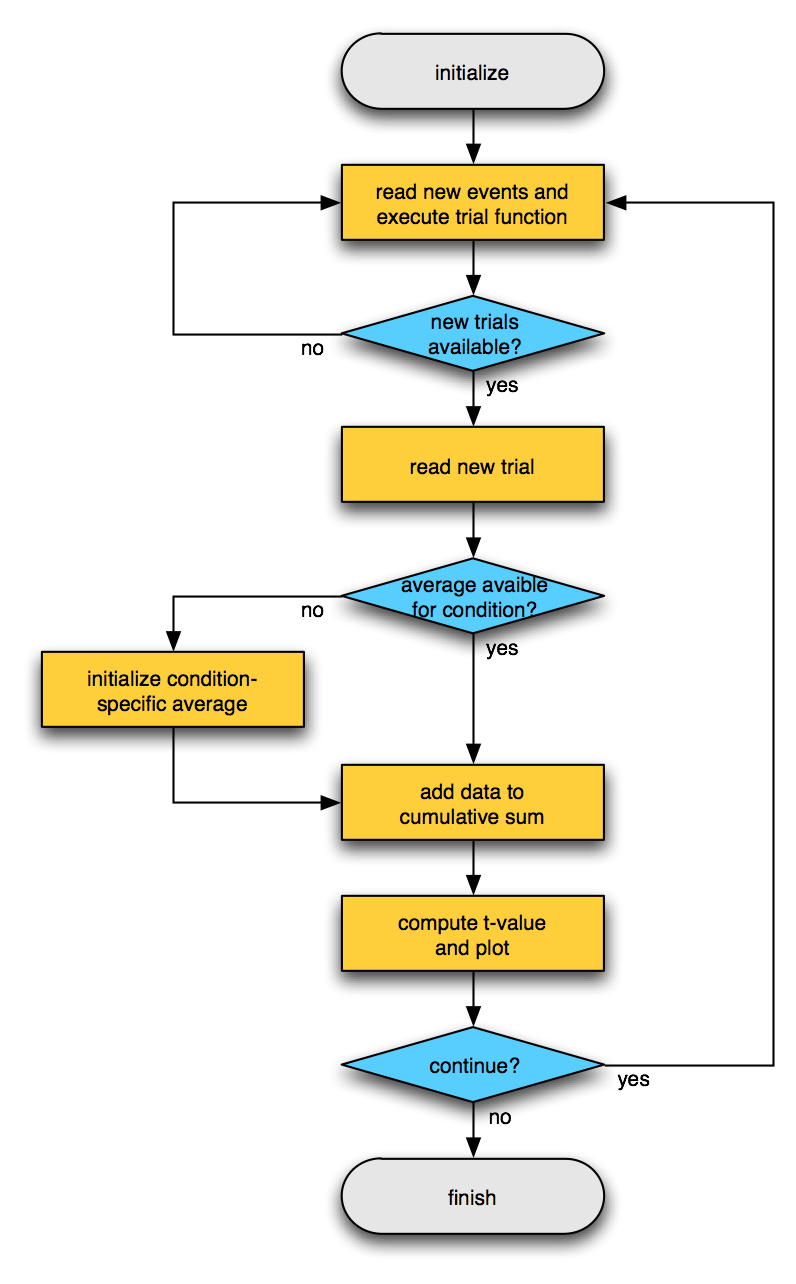Tags: example realtime

# Example real-time selective average

## Flowchart## MATLAB code

``````function ft_realtime_selectiveaverage(cfg)

% FT_REALTIME_SELECTIVEAVERAGE is an example realtime application for online
% averaging of the data. It should work both for EEG and MEG.
%
% Use as
%   ft_realtime_selectiveaverage(cfg)
% with the following configuration options
%   cfg.channel    = cell-array, see FT_CHANNELSELECTION (default = 'all')
%   cfg.trialfun   = string with the trial function
%
% The source of the data is configured as
%   cfg.dataset       = string
% or alternatively to obtain more low-level control as
%   cfg.datafile      = string
%   cfg.headerfile    = string
%   cfg.eventfile     = string
%   cfg.dataformat    = string, default is determined automatic
%   cfg.headerformat  = string, default is determined automatic
%   cfg.eventformat   = string, default is determined automatic
%
% To stop the realtime function, you have to press Ctrl-C

% Copyright (C) 2008, Robert Oostenveld
%
% Subversion does not use the Log keyword, use 'svn log `<filename>` or 'svn -v log | less' to get detailled information

% set the default configuration options
if ~isfield(cfg, 'dataformat'),     cfg.dataformat = [];      end % default is detected automatically
if ~isfield(cfg, 'headerformat'),   cfg.headerformat = [];    end % default is detected automatically
if ~isfield(cfg, 'eventformat'),    cfg.eventformat = [];     end % default is detected automatically
if ~isfield(cfg, 'channel'),        cfg.channel = 'all';      end
if ~isfield(cfg, 'bufferdata'),     cfg.bufferdata = 'last';  end % first or last

% translate dataset into datafile+headerfile
cfg = ft_checkconfig(cfg, 'dataset2files', 'yes');
cfg = ft_checkconfig(cfg, 'required', {'datafile' 'headerfile'});

% ensure that the persistent variables related to caching are cleared
% start by reading the header from the realtime buffer

% define a subset of channels for reading
cfg.channel = channelselection(cfg.channel, hdr.label);
chanindx    = match_str(hdr.label, cfg.channel);
nchan       = length(chanindx);
if nchan==0
error('no channels were selected');
end

prevSample = 0;
count      = 0;

% initialize the timelock cell-array, each cell will hold the average in one condition
timelock = {};

%%%%%%%%%%%%%%%%%%%%%%%%%%%%%%%%%%%%%%%%%%%%%%%%%%%%%%%%%%%%%%%%%%%%%%%%%%%%%%%%
% this is the general BCI loop where realtime incoming data is handled
%%%%%%%%%%%%%%%%%%%%%%%%%%%%%%%%%%%%%%%%%%%%%%%%%%%%%%%%%%%%%%%%%%%%%%%%%%%%%%%%
while true

% determine latest header and event information
event     = read_event(cfg.dataset, 'minsample', prevSample+1);  % only consider events that are later than the data processed sofar
hdr       = read_header(cfg.dataset, 'cache', true);             % the trialfun might want to use this, but it is not required
cfg.event = event;                                               % store it in the configuration, so that it can be passed on to the trialfun
cfg.hdr   = hdr;                                                 % store it in the configuration, so that it can be passed on to the trialfun

% evaluate the trialfun, note that the trialfun should not re-read the events and header
fprintf('evaluating ''%s'' based on %d events\n', cfg.trialfun, length(event));
trl = feval(cfg.trialfun, cfg);

% the code below assumes that the 4th column of the trl matrix contains the condition index
% set the default condition to one if no condition index was given
if size(trl,2)<4
trl(:,4) = 1;
end

fprintf('processing %d trials\n', size(trl,1));

for trllop=1:size(trl,1)

begsample = trl(trllop,1);
endsample = trl(trllop,2);
offset    = trl(trllop,3);
condition = trl(trllop,4);

% remember up to where the data was read
prevSample  = endsample;
count       = count + 1;
fprintf('processing segment %d from sample %d to %d, condition = %d\n', count, begsample, endsample, condition);

% read data segment from buffer
dat = ft_read_data(cfg.datafile, 'header', hdr, 'begsample', begsample, 'endsample', endsample, 'chanindx', chanindx, 'checkboundary', false);

%%%%%%%%%%%%%%%%%%%%%%%%%%%%%%%%%%%%%%%%%%%%%%%%%%%%%%%%%%%%%%%%%%%%%%%%%%%%%%%%
% from here onward it is specific to the processing of the data
%%%%%%%%%%%%%%%%%%%%%%%%%%%%%%%%%%%%%%%%%%%%%%%%%%%%%%%%%%%%%%%%%%%%%%%%%%%%%%%%

% put the data in a fieldtrip-like raw structure
data.trial{1} = dat;
data.time{1}  = offset2time(offset, hdr.Fs, endsample-begsample+1);
data.label    = hdr.label(chanindx);
data.hdr      = hdr;
data.fsample  = hdr.Fs;

% apply some preprocessing options
data.trial{1} = preproc_baselinecorrect(data.trial{1});

if length(timelock)<condition || isempty(timelock{condition})
% this is the first occurence of this condition, initialize an empty timelock structure
timelock{condition}.label   = data.label;
timelock{condition}.time    = data.time{1};
timelock{condition}.avg     = [];
timelock{condition}.var     = [];
timelock{condition}.dimord  = 'chan_time';
nchans   = size(data.trial{1}, 1);
nsamples = size(data.trial{1}, 2);
% the following elements are for the cumulative computation
timelock{condition}.n   = 0;                          % number of trials
timelock{condition}.s   = zeros(nchans, nsamples);    % sum
timelock{condition}.ss  = zeros(nchans, nsamples);    % sum of squares
end

% add the new data to the accumulated data
timelock{condition}.n  = timelock{condition}.n  + 1;
timelock{condition}.s  = timelock{condition}.s  + data.trial{1};
timelock{condition}.ss = timelock{condition}.ss + data.trial{1}.^2;

% compute the average and variance on the fly
timelock{condition}.avg = timelock{condition}.s ./ timelock{condition}.n;
timelock{condition}.var = (timelock{condition}.ss - (timelock{condition}.s.^2)./timelock{condition}.n) ./ (timelock{condition}.n-1);

%%%%%%%%%%%%%%%%%%%%%%%%%%%%%%%%%%%%%%%%%%%%%%%%%%%%%%%%%%%%%%%%%%%%%%%%%%%%%%%%
% from here onward additional processing of the selective averages could be done
% as an example here the ERP of each condition is plotted in its own figure
%%%%%%%%%%%%%%%%%%%%%%%%%%%%%%%%%%%%%%%%%%%%%%%%%%%%%%%%%%%%%%%%%%%%%%%%%%%%%%%%

% compute the t-score versus zero by dividing the average by the standard error of mean
tscore = timelock{condition}.avg ./ (sqrt(timelock{condition}.var)./(timelock{condition}.n - 1));

figure(condition)
plot(timelock{condition}.time, tscore);
title(sprintf('condition %d, ntrials = %d', condition, timelock{condition}.n));

% force MATLAB to redraw the figure
drawnow

end % looping over new trials
end % while true
``````